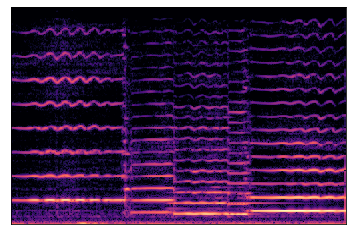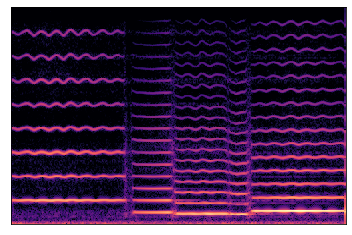The discriminator I used is similar to what was described in https://arxiv.org/abs/2012.07267 and https://arxiv.org/abs/1910.06711. The input to the discriminator is f0, amplitudes, harmonic distribution, and the noise magnitudes, conditioned on the conditioning signals (note expression controls). The discriminator uses three identical discriminator networks on three scales of data: the original, average pooled 2x, and average pooled 4x. Each discriminator network uses 4 blocks and each block consists of two 1x3 conv blocks with residual connections and LeakyRelu (similar to the one used in https://arxiv.org/abs/2012.07267). The discriminator only applies on the output other than f0, the f0 is still learned and generated by an autoregressive RNN. The discriminator training objective is Least Square GAN, and the generator training objective is Least Square GAN loss, reconstruction loss (spectral loss), f0 cross entropy loss and feature matching loss of the discriminator feature map.

The best model that I tuned now uses a smaller learning rate than the generator (1e-4 vs. 3e-4) and blocks the gradient from discriminator to f0 autoregressive RNN. I also tried adding noise to the generator by using noise as input to the dilated conv, and use the conditioning vector as conditioning. It has similar results, for which I can't really tell the difference.

Here are the results: It can be heard from the samples that it is now more similar to the timbre of the original recording. And looking from the harmonic distribution and noise magnitudes plots, there is no more over-smoothing.

# Original recording:¶

In :
import utils.audio_io

In :
wav = utils.audio_io.load_audio(r'/data/ddsp-experiment/logs/logs/ref.wav', 16000)
plot_spec(wav, hp.sample_rate, title='')# Audio sample of old model that have oversmoothing problem:¶

In :
wav = utils.audio_io.load_audio(r'/data/ddsp-experiment/logs/logs/pred_cnn.wav', 16000)
plot_spec(wav, hp.sample_rate, title='')# Audio sample of GAN model:¶

In :
plot_spec(outputs['midi_audio'].numpy(), sample_rate)